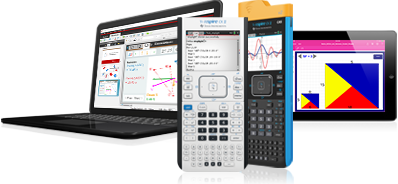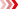Algebra I
Find activities that support your lesson plans

Challenge students to use and think about how technology can be used to model, analyze and explain math with standards-aligned activities in eight algebra I topics.

Activities can be used as is or edited to support specific objectives, align with popular text books and include technology tips to help you focus the learning and address student misconceptions.# Featured Activities

## Multiple Representations

This lesson involves the concept of interpreting slope as a rate of change in the context of a real-world problem situation.## Exploring Domain and Range

This lesson involves identifying a set of x-values in both symbols and words, identifying the set of x-values used in generating the function as the domain of the function, and identifying the set of y-values used in generating the function as the range of the function.## Linear Inequalities in Two Variable

This lesson involves the basics of graphing linear inequalities. As a result, students will formulate the basic concepts of graphing inequalities and graph systems of inequalities.